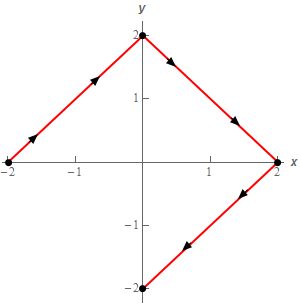Paul's Online Notes
Home / Calculus III / Line Integrals / Fundamental Theorem for Line Integrals
Show Mobile Notice Show All Notes Hide All Notes
Mobile Notice
You appear to be on a device with a "narrow" screen width (i.e. you are probably on a mobile phone). Due to the nature of the mathematics on this site it is best views in landscape mode. If your device is not in landscape mode many of the equations will run off the side of your device (should be able to scroll to see them) and some of the menu items will be cut off due to the narrow screen width.

### Section 16.5 : Fundamental Theorem for Line Integrals

1. Evaluate $$\displaystyle \int\limits_{C}{{\nabla f\centerdot d\vec r}}$$ where $$f\left( {x,y} \right) = {x^3}\left( {3 - {y^2}} \right) + 4y$$ and C is given by $$\vec r\left( t \right) = \left\langle {3 - {t^2},5 - t} \right\rangle$$ with $$- 2 \le t \le 3$$. Solution
2. Evaluate $$\displaystyle \int\limits_{C}{{\nabla f\centerdot d\vec r}}$$ where $$f\left( {x,y} \right) = y{{\bf{e}}^{{x^{\,2}} - 1}} + 4x\sqrt y$$ and C is given by $$\vec r\left( t \right) = \left\langle {1 - t,2{t^2} - 2t} \right\rangle$$ with $$0 \le t \le 2$$. Solution
3. Given that $$\displaystyle \int\limits_{C}{{\vec F\centerdot d\vec r}}$$ is independent of path compute $$\displaystyle \int\limits_{C}{{\vec F\centerdot d\vec r}}$$ where C is the ellipse given by $$\displaystyle \frac{{{{\left( {x - 5} \right)}^2}}}{4} + \frac{{{y^2}}}{9} = 1$$ with the counter clockwise rotation. Solution
4. Evaluate $$\displaystyle \int\limits_{C}{{\nabla f\centerdot d\vec r}}$$ where $$f\left( {x,y} \right) = {{\bf{e}}^{x\,y}} - {x^2} + {y^3}$$ and C is the curve shown below.Solution